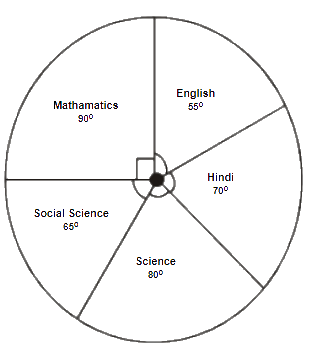# Ex.5.2 Q4 Data Handling Solutions - NCERT Maths Class 8

Go back to  'Ex.5.2'

## Question

The adjoining pie chart gives the marks scored in an examination by a student in Hindi, English, Mathematics, Social Science and Science. If the total marks obtained by the students were $$540,$$ answer the following questions:(i) In which subject did the student score $$105$$ marks?
(Hint: for $$540$$ marks, the central angle$$= 360^\circ$$ a So, for $$105$$ marks, what is the central angle?)

(ii) How many more marks were obtained by the student in Mathematics than in Hindi?

(iii) Examine whether the sum of the marks obtained in Social Science and Mathematics is more than that in Science and Hindi.
(Hint: Just study the central angles)

Video Solution
Data Handling
Ex 5.2 | Question 4

## Text Solution

What is known?

(i) Pie chart describing the marks scored in an examination by a student in Hindi, English, Mathematics, Social Science and Science.

(ii) Total marks obtained by the students are $$540$$

What is unknown?

(i)  The subject in which the student scored $$105$$ marks

(ii)  Number of more marks scored by the student in Mathematics than in Hindi

(iii) Whether the sum of the marks obtained in Social Science and Mathematics is more than that in Science and Hindi.

Reasoning:

A circle graph or pie chart shows the relationship between a whole and its parts. Sum of the total angles in a pie chart is equal to $$360.$$

Steps:

 Subject Central Angle Marks obtained Mathematics $$90^\circ$$ \begin{align}\frac{{{{90}^{\circ}}}}{{{{360}^{\rm{o}}}}} \times 540 = 135\end{align} Social Science $$65^\circ$$ \begin{align}\frac{{{{65}^\circ}}}{{{{360}^{\circ}}}} \times 540 = 97.5\end{align} Science $${80^{\circ}}$$ \begin{align}\frac{{{{80}^{\circ}}}}{{{{360}^{\circ}}}} \times 540 = 120\end{align} Hindi $$70^\circ$$ \begin{align}\frac{{{{70}^{\circ}}}}{{{{360}^{\circ}}}} \times 540 = 105\end{align} English $$55^\circ$$ \begin{align}\frac{{{{55}^{\circ}}}}{{{{360}^{\circ}}}} \times 540 = 82.5\end{align}

(i) The student scored $$105$$ marks in Hindi.

(ii) Marks obtained in Mathematics $$= 135$$

Marks obtained in Hindi $$= 105$$

Difference $$= 135 – 105 = 30$$

Thus, $$30$$ more marks were obtained by the student in Mathematics than in Hindi.

(iii) The sum of marks in Social Science and Mathematics $$= 97.5 + 135 = 232.5$$

The sum of marks in Science and Hindi $$= 120 + 105 = 225$$

Yes, the sum of the marks in Social Science and Mathematics is more than that in Science and Hindi.

Learn from the best math teachers and top your exams

• Live one on one classroom and doubt clearing
• Practice worksheets in and after class for conceptual clarity
• Personalized curriculum to keep up with school